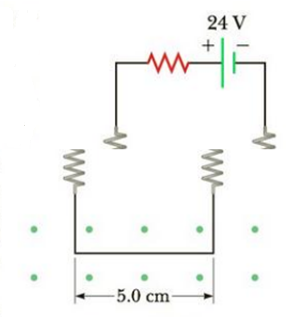Chapter 19, Problem 74AP

Chapter
Section
Textbook Problem

A straight wire of mass 10.0 g and length 5.0 cm is suspended from two identical springs that, in turn, form a closed circuit (Fig. P19.74). The springs stretch a distance of 0.50 cm under the weight of the wire. The circuit has a total resistance of 12 Ω. When a magnetic field directed out of the page (indicated by the dots in the Figure) is turned on, the springs are observed to stretch an additional 0.30 cm. What is the strength of the magnetic field? (The upper portion of the circuit is fixed.)Figure P19.74

To determine
The strength of the magnetic field.

Explanation

Given Info:

The mass of the straight wire is 10.0g . The length of the wire is 5.0cm . The wire is suspended from two identical springs which is a part of a closed circuit. The springs stretch a distance of 0.50cm under the weight of the wire.

Explanation:

The total resistance of the circuit is 12Ω . The direction of the magnetic field is out of the page. When the magnetic field is turned on the extra stretch in the springs is 0.30cm .

From Hooke’s law the force constant of a spring is given by,

k=Fx

• F is the applied force
• x is the stretch in the springs on the application of the force

Applying Hooke’s law to the initial case where the spring is elongated only due to the mass of the wire so the force is the gravitational force,

k=mgx1       (1)

• m is the mass of the wire
• x1 is the elongation only due to the mass of the wire

When the magnetic field is turned on there will be an additional force due to the magnetic field.

Applying Hooke’s law when the magnetic field is turned on,

k=Fm+mgx1+x2       (2)

• x2 is the extra elongation in the spring when the magnetic field is turned on
• Fm is the magnetic force

From (1) and (2),

Fm+mgx1+x2=mgx1

The magnetic force will be,

Fm=mgx1(x1+x2)mg       (3)

Since the angle between the magnetic field and current direction is perpendicular to each other, the magnetic force will be equal to,

Fm=BIL       (4)

• I is the current
• L is the length if the wire
• B is the magnetic field

Combining (3) and (4),

BIL=mgx1(x1+x2)mg

The magnetic field will be,

B=1IL(mgx1(x1+x2)mg)

From ohms law the current in the wire will be,

I=VR       (5)

• V is the voltage supplied
• R is the resistance of the circuit

Using (5) the magnetic field will be,

B=RVL(mgx1(x1+x2)mg)

Substitute 10

Still sussing out bartleby?

Check out a sample textbook solution.

See a sample solution

The Solution to Your Study Problems

Bartleby provides explanations to thousands of textbook problems written by our experts, many with advanced degrees!

Get Started

What does density describe?

An Introduction to Physical Science

8-13 Define (a) an Arrhenius acid and (b) an Arrhenius base.

Introduction to General, Organic and Biochemistry

Why did the Copernican hypothesis win gradual acceptance?

Horizons: Exploring the Universe (MindTap Course List)